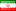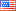••# what is Flow Computer?Flow computer is used to measure the flow of gases or liquids. Using digital and analog inputs, it receives flowmeter values, temperature sensors, and gas volume and measures the exact flow rate by using complex mathematical equations. The flow meter signal can be a type of pressure difference (such as an orifice plate) or a direct flow rate (such as a turbine or ultrasonic). In the type of pressure difference, it is necessary to calculate the mathematical equations of Bernoulli, Boyle and Reynolds to calculate the flow rate.According to the general law of complete gases and also because there is no complete gas in nature, the most important thing about measuring the gas flow is to consider the PTZ correction. The PTZ correction converts the measured amount of gas under normal conditions to the reference conditions of temperature (T) and pressure (P) (PT). The Z parameter is used because of the non-linear density of gases called the compressibility coefficient. This value actually denotes the difference between the actual volume of the gas and its nominal volume at a constant pressure and temperature.

The next point in measuring the flow is normalizing the read value. There are two methods of Normal and standard, that both of them are commonly used in the industry. The difference between these two methods is the reference temperature value considered for normalization (zero degrees for Normal and 15.6 degrees for Standard). To get the exact value you need to use some standards. Currently the main standards are AGA3, AGA8 and NX19. The NX19 standard is used to calculate the gas compressibility coefficient (term Z in PTZ).

PR56-18F Flow Computer

Flow Computer Designed by Arman Fara Pajoohan Company uses the values of pressure, pressure and temperature difference as well as PTZ correction to measure and record the flow rate according to the existing high precision standards. The PR-5618F also has all the features of a recorder in addition to measuring the flow. Unlimited data recording, displaying measured values in graphs, bar graphs and digital, PID controller, universal input, relay output, SSR and Open Collector, and advanced formulating are just a few of the features implemented on this computer.Other features of this device is its very precise totalizer. This totalizer can measure the desired total values at user selectable intervals. Usage amount is selectable on 8-hour intervals (for work shifts), 24-hour as well as total use after zeroing the counter on the device.

The device is currently installed in Gilan Combined Cycle Power Plant, Shahid Beheshti Power Plant, Tehran Besat Power Plant, and Mobarakeh Steel Company.

Here are some of the PR-5618 Flow computer Features:

• Colored touch screen
• Unlimited storage of input signal data
• Ability to review data and transfer to computer
• Designed according to NX19, AGA, ISO standards
• Calculate the Z compressibility coefficient based on the gas analysis values
• Ability to connect directly to a variety of pressure difference flowmeters
• Ability to display different data: graph, digital, bar graph, etc.
• 6 isolated 24-bit universal input channels
• Capable of connecting all types of thermocouples, thermos resistors, mA, voltage, frequency
• 8 Customizable Output Contact Relays
• 2 Programmable analog output with PID controller
• RS485 serial port with Modbus protocol
• Very accurate totalizer
• Designed in 2 sizes of industry standard